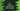# C# program to check if a string starts with a number or not## How to check if a string starts with a number in C#:

In this post, we will learn how to check if a string starts with a number or not in C#. For that, we need to get the first character of the string. Then, we need to check if it is a number or not. That’s it.

String is immutable in C#. But, we can access the characters of a string using index. index starts from 0 and we can get the first character of the string using 0 as the index.

Then, we can use Char.IsDigit method to check if a character is number or not.

### Char.IsDigit(char ch):

IsDigit method is defined as below:

``public static bool IsDigit(char ch);``

This is a static method and it accepts one character as its parameter. Here, ch is the unicode character to check if it is a digit or not.

It returns one boolean value. true if it is a decimal value, else false.

This method can be used to check if the first character is a number or not of a string.

Below is the complete program:

``````using System;

public class Program
{
public static void Main()
{
string str1 = "hello world";
string str2 = "123 hello world";

Console.WriteLine(Char.IsDigit(str1));
Console.WriteLine(Char.IsDigit(str2));
}
}``````

Here,

• str1 and str2 are two strings.
• We are getting the first character of the string by using the index 0.

It will print the below output:

``````False
True``````

### Char.IsDigit(String, Int32):

This method is defined as below:

``public static bool IsDigit(string str, int i);``

This is a static method and we can pass one string to this method and one index. It checks if the character at position i of the string str is digit or not.

Let’s write the above program using this method:

``````using System;

public class Program
{
public static void Main()
{
string str1 = "hello world";
string str2 = "123 hello world";

Console.WriteLine(Char.IsDigit(str1, 0));
Console.WriteLine(Char.IsDigit(str2, 0));
}
}``````

It will print:

``````False
True ``````